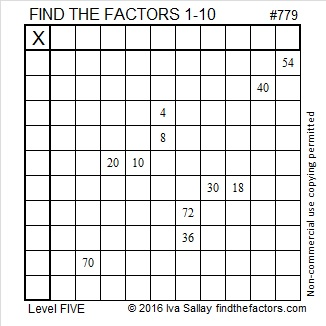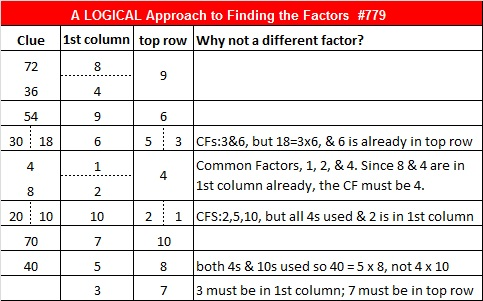# Can You See How 779’s Factor Pairs Are Hiding in Some Pythagorean Triples?

• 779 is a composite number.
• Prime factorization: 779 = 19 x 41
• The exponents in the prime factorization are 1 and 1. Adding one to each and multiplying we get (1 + 1)(1 + 1) = 2 x 2 = 4. Therefore 779 has exactly 4 factors.
• Factors of 779: 1, 19, 41, 779
• Factor pairs: 779 = 1 x 779 or 19 x 41
• 779 has no square factors that allow its square root to be simplified. √779 ≈ 27.91057.Those factor pairs are hiding in some Pythagorean triples. Scroll down to read how, but first here’s today’s puzzle:Print the puzzles or type the solution on this excel file: 10-factors-2016

————————————–

And now some Pythagorean triple number theory using 779 as an example:

The factors of 779 are very well hidden in five Pythagorean triples that contain the number 779. Here’s how: 779 has two factor pairs:  19 x 41 and 1 x 779. Those factor pairs show up in some way in each of the calculations for these 779 containing Pythagorean triples:

1. 171-760-779 which is 19 times each number in 9-40-41.
2. 779-303420-303421, a primitive calculated from 779(1); (779² – 1²)/2; (779² + 1²)/2.
3. 779-7380-7421 which is 41 times each number in 19-180-181.
4. 779-15960-15979 which is 19 times each number in 41-840-841.
5. 660-779-1021, a primitive calculated from (41² – 19²)/2; 19(41); (41² + 19²)/2.

Being able to find whole numbers that satisfy the equation a² + b² = c² is one reason why finding factors of a number is so worth it. ANY factor pair for numbers greater than 2 will produce at least one Pythagorean triple that satisfies a² + b² = c². The more factor pairs a number has, the more Pythagorean triples will exist that contain that number. 779 has only two factor pairs so there are a modest number of 779 containing Pythagorean triples. All of its factors are odd so it was quite easy to find all of the triples. Here’s a brief explanation on how each triple was found:

1. 799 has one prime factor that has a remainder of 1 when divided by 4. That prime factor, 41, is therefore the hypotenuse of a primitive Pythagorean triple. When the Pythagorean triple is multiplied by the other half of 41’s factor pair, 19, we get a Pythagorean triple in which 779 is the hypotenuse.
2. Every odd number greater than 1 is the short leg of a primitive Pythagorean triple. To find that primitive for a different odd number, simply substitute the desired odd number in the calculation in place of 779.
3. Because every odd number greater than 1 is the short leg of a primitive Pythagorean triple, 19(1); (19² – 1²)/2; (19² + 1²)/2 generates the primitive triple (19-180-181). Multiplying each number in that triple by the other half of 19‘s factor pair, 41, produces a triple with 779 as the short leg.
4. Because every odd number greater than 1 is the short leg of a primitive Pythagorean triple, 41(1); (41² – 1²)/2; (41² + 1²)/2 generates the primitive triple (41-840-841). Multiplying each number in that triple by the other half of 41‘s factor pair, 19, produces a triple with 779 as the short leg.
5. Since factor pair 19 and 41 have no common prime factors, the formula (41² – 19²)/2; 19(41); (41² + 19²)/2 produces another primitive triple 660-779-1021. If they did have common factors, the factor pair would still produce a triple, but it would not be a primitive one.

Here’s some other interesting facts about the number 779:

779 is the sum of eleven consecutive prime numbers:

47 + 53 + 59 + 61 + 67 + 71 + 73 + 79 + 83 + 89 + 97 = 779.

779 can also be written as the sum of three squares six different ways:

• 27² + 7² + 1² = 779
• 27² + 5² + 5² = 779
• 23² + 15² + 5² = 779
• 23² + 13² + 9² = 779
• 21² + 17² + 7² = 779
• 21² + 13² + 13² = 779

Finally, the table below shows some logical steps that could be used to solve Puzzle #779:## 2 thoughts on “Can You See How 779’s Factor Pairs Are Hiding in Some Pythagorean Triples?”

1.davescarthin

Aaaargh! …the 41 and 19 effects arouse the “I could never do mathematics” feeling, so common among the population, even (??) in ME!

•ivasallay

Really? You’re so brilliant creating tessellations from numbers. Nevertheless, thank you so much for the feedback.

Your comment caused me to rearrange the items in this post from easiest to understand to most difficult to understand. I trust someone out there will find even the number theory interesting AND be able to understand it, too.

This site uses Akismet to reduce spam. Learn how your comment data is processed.